Rg-Calculator for Platonic Solids (SAS)

Enter the value (a) for either the edge length, circum-radius, in-sphere-radius, mid-radius, surface or volume, respectively, of a Tetrahedron / Hexahedron (Cube) / Octahedron / Dodecahedron / Icosahedron. Their radius of gyration (Rg) of the solid, of the surface (faces) and of the perimeter (edges) will be calculated together with the volume, the surface (sum of all face areas) and the perimeter (sum of all edge lengths), as well as for comparison also for a sphere with the value (=a) for the selected parameter (sphere radius r, surface or volume, respectively). Units are arbitrary.

 Parameter Tetrahedron Hexahedron Octahedron Dodecahedron Icosahedron Sphere Shape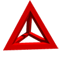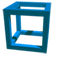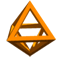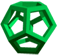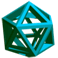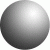edge-length a in-sphere r mid-sphere r circum-sphere r Perimeter Surface Volume Rg (edges) Rg (surface) Rg (solid)